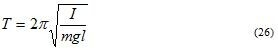# Simple Harmonic Motion

### (B) Simple pendulum

• Simple pendulum consists of a point mass suspended by inextensible weightless string in a uniform gravitational field.
• Simple pendulum can be set into oscillatory motion by pulling it to one side of equilibrium position and then releasing it.
• In case of simple pendulum path ot the bob is an arc of a circle of radius l, where l is the length of the string.
• We know that for SHM F=-kx and here x is the distance measured along the arc as shown in the figure below.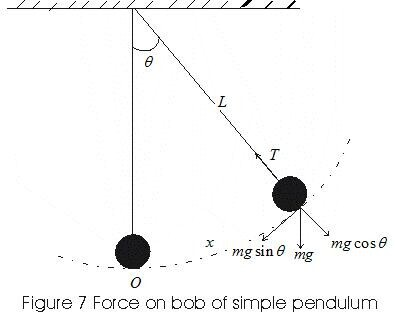• When bob of the simple pendulum is displaced from its equilibrium position O and is then released it begins to oscillate.
• Suppose it is at P at any instant of time during oscillations and θ be the angle subtended by the string with the vertical.
• mg is the force acting on the bob at point P in vertically downward direction.
• Its component mgcosθ is balanced by the tension in the string and its tangential component mgsinθ directs in the direction opposite to increasing θ .
• Thus restoring force is given by
F=-mgsinθ               (19)
• The restoring force is proportional to sinθ not to The restoring force is proportional to sinθ, so equation 19 does not represent SHM.
• If the angle θ is small such that sinθ very narly equals θ then above equation 19 becomes
F=-mgθ
since x=lθ then,
F=-(mgx)/l
where x is the displacement OP along the arc. Thus,
F=-(mg/l)x               (20)
• From above equation 20 we see that restoring forcr is proportional to coordinate for small displacement x , and the constant (mg/l) is the force constant k.
• Time period of a simple pendulum for small amplitudes is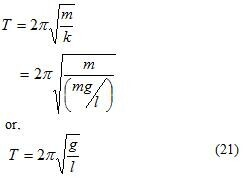• Corresponding frequency relations are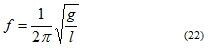and angular frequency
ω=√(g/l)          (23)
• Notice that the period of oscillations is independent of the mass m of the pendulumand for small oscillations pperiod of pendulum for given value of g is entirely determined by its length.

### (c) The compound pendulum

• Compound pendulum is a rigid body of any shape, capable of oscillating about a horizontal axis passing through it.
• Figure below shows vertical section of rigid body capable of oscillating about the point A.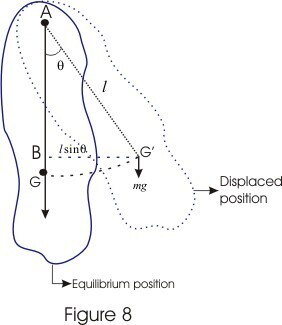• Distance l between point A and the centre of gravity G is called length of the pendulum.
• When this compound pendulum is given a small angulr displacement θ and is then released it begins to oscillate about point A.
• At angular displacement θ its center of gravity now takes new position at G'.
• Weight of the body and its reaction at the support constitute a reactive couple or torque given by
τ=-mg G'B
=-mglsinθ
(24)
• Equation 24 gives restoring couple which tends to bring displaced body to its original position.
• If α is the angular acceleration produced in this body by the couple and I is the moment of inertia of body about horizontal axis through A then the couple is
Iα=-mglsinθ
if θ is very small then we can replace sinθ≅θ, so that
α=-(mgl/I)θ          (25)
• From above equation (25) we se that pendulum is executing Simple Harmonic Motion with time period